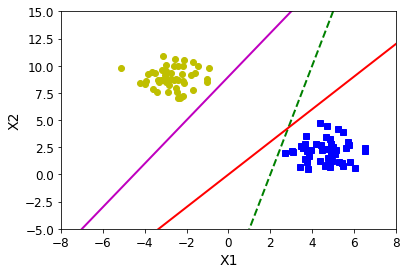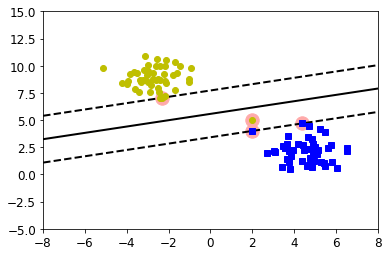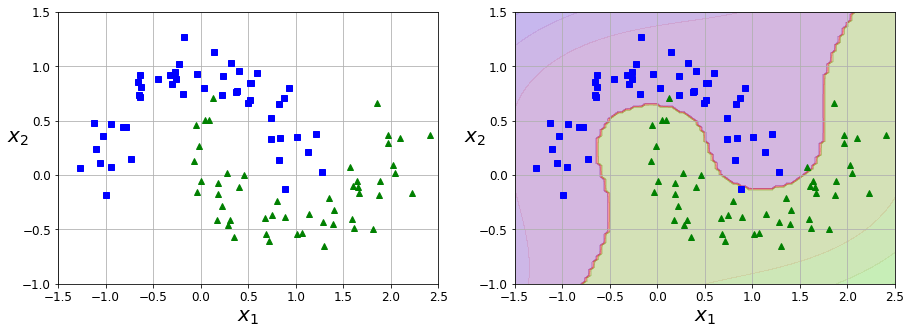# What is a support vector machine (SVM)?

This article is part of Demystifying AI, a series of posts that (try to) disambiguate the jargon and myths surrounding AI.

Support vector machine (SVM) is a type of machine learning algorithm that can be used for classification and regression tasks. They build upon basic ML algorithms and add features that make them more efficient at various tasks.

Support vector machines can be used in a variety of tasks, including anomaly detection, handwriting recognition, and text classification. Because of their flexibility, high performance, and compute efficiency, SVMs have become a mainstay of machine learning and an important addition to the ML engineer’s toolbox.

## Support vectors

Support vector machines are among supervised machine learning algorithms, which means they need to be trained on labeled data. To understand support vector machines, consider the following dataset, composed of a set of points from two different classes (yellow and blue).

A machine learning model can learn to classify the points into the two classes through linear separation. However, you can create many different ML models to separate the two classes, and not all of them are of equal value.Many different machine learning models can separate classes of data, but not all of them provide good performance

For example, if you draw the line too close to one of the classes, your model will become sensitive to small deviations from the norm on that class. Therefore, if a new point is a bit farther from the cluster used during training, the ML model will misclassify it.Bad ML models will be too sensitive to small changes and can easily misclassify unseen data

SVMs use “support vectors” to find optimal solutions to classification problems. Support vectors (dashed lines in the figure below) are at equal distance of the main classification line (solid line). By placing the support vectors on the edge instances of each class, the SVM creates an ML model that is robust to unseen data that do not closely fit the training examples.SVMs use “support vectors” to find the optimal model that creates the most distance from the edge of classes

## Hard and soft margins

SVMs can have hard and soft margins. If you think as support vectors and the main classification line as a street, a hard margin SVM will try to place all instances off or at the edge of the street. This is not a bad strategy if the instances of all classes are divided into neat clusters.

But if the data contains outliers, then the SVM will draw a bad boundary. And if there is overlap between the classes, then a hard-margin SVM won’t work at all.

To address these problems, SVMs support “soft margins,” a hyperparameter that can be adjusted before training the model. Soft margins allow a number of instances to violate the support vector boundaries to choose a better classification line. The lower the soft margin number (usually specified by the Greek letter zeta, or “C” in programming libraries), the more boundary violations the model allows and the wider the SVM street becomes. Choosing the right soft margin value will ensure the SVM will have the right balance between clean boundaries and generalizability. In the example below, even though one outlier in the training data has been misclassified, the soft margin has resulted in a better classification line.Soft margins create a balance between margin violations and generalizability

## Kernel tricks

In some datasets, the classes are not “linearly separable,” which means they can’t be separated with a straight line. In such cases, ML engineers use “kernel tricks,” mathematical transformations that can make the data linearly separable.

On popular kernel trick is the “polynomial kernel,” which adds more dimensions to the dataset to make it linearly separable. For example, in left figure below, the classes in the dataset are interspersed on a straight line. By adding an extra dimension to the dataset (e.g., x^2), we can rearrange the datapoints in a way that makes them linearly separable (right figure).

Similarly, the polynomial kernel can be applied to other datasets where the boundaries between classes are more complicated. For example, the dataset below was generated with the make_moons() function of Python’s Scikit-Learn machine learning library. Evidently, the two classes are not linearly separable. But an SVM with a third-degree polynomial kernel can clearly separate the two classes with a smooth boundary (right figure).The kernel trick can help SVMs classify classes that are not linearly separable

Another popular kernel is the Gaussian RBF kernel, which uses the radial basis function to measure the distance between different datapoints and make the classes linearly separable. SVM comes with many other kernel tricks that can be used for different applications.

## Applications of support vector machines

Despite the advent of more advanced machine learning algorithms such as deep neural networks, support vector machines remain popular because of their fast training time, low compute requirements, and ability to learn with fewer training examples.

For some computer vision tasks, SVMs can perform with accuracy that rivals neural networks at a fraction of the computational costs. A popular application is face detection, where the SVM model draws a bounding box around faces in images or video feeds. Such models are lightweight and can often be directly deployed on cameras with small computing capacity to avoid sending and processing data in the cloud. SVMs can also be used for image classification and handwriting recognition.

SVMs can also be used for some applications of natural language processing (NLP) such as topic and intent classification, spam detection, and sentiment analysis. After being transformed into numerical values through techniques such as bag of words, text data can be used to train support vector machines for the target task.

Support vector machines are a powerful tool that every machine learning engineer should consider when tackling a new problem.

This site uses Akismet to reduce spam. Learn how your comment data is processed.